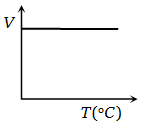In the adjoining figure, various isothermals are shown for a real gas. Then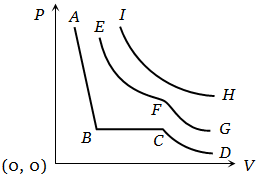1. EF represents liquification

2. CB represents liquification

3. HI represents the critical temperature

4. AB represents gas at a high temperature

High Yielding Test Series + Question Bank - NEET 2020

Difficulty Level:

From the following P-T graph what interference can be drawn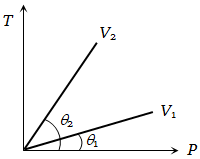1.

2.

3.

4. None of the above

High Yielding Test Series + Question Bank - NEET 2020

Difficulty Level:

PV versus T graph of equal masses of ${\mathrm{H}}_{2}$$\mathrm{He}$ and ${\mathrm{O}}_{2}$ is shown in fig. Choose the correct alternative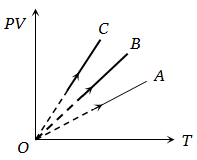1. C corresponds to ${\mathrm{H}}_{2}$, B to $\mathrm{He}$ and A to ${\mathrm{O}}_{2}$

2. A corresponds to $\mathrm{He}$, B to ${\mathrm{H}}_{2}$ and C to ${\mathrm{O}}_{2}$

3. A corresponds to $\mathrm{He}$, B to ${\mathrm{O}}_{2}$ and C to ${\mathrm{H}}_{2}$

4. A corresponds to ${\mathrm{O}}_{2}$, B to ${\mathrm{H}}_{2}$ and C to ${\mathrm{H}}_{2}$

High Yielding Test Series + Question Bank - NEET 2020

Difficulty Level:

Pressure versus temperature graph of an ideal gas at constant volume V of an ideal gas is shown by the straight line A. Now mass of the gas is doubled and the volume is halved, then the corresponding pressure versus temperature graph will be shown by the line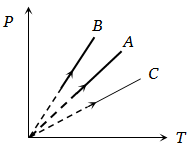1. A

2. B

3. C

4. None of these

High Yielding Test Series + Question Bank - NEET 2020

Difficulty Level:

Two different isotherms representing the relationship between pressure P and volume V at a given temperature of the same ideal gas are shown for masses ${\mathrm{m}}_{1}$ and ${\mathrm{m}}_{2}$ of the gas respectively in the figure given, then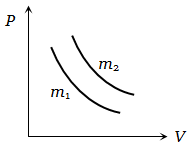1.

2.

3.

4. None of these

High Yielding Test Series + Question Bank - NEET 2020

Difficulty Level:

Two different masses m and 3m of an ideal gas are heated separately in a vessel of constant volume, the pressure P and absolute temperature T, graphs for these two cases are shown in the figure as A and B. The ratio of slopes of curves B to A is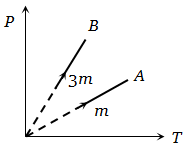1.  3 : 1

2.  1 : 3

3.  9 : 1

4.  1 : 9

High Yielding Test Series + Question Bank - NEET 2020

Difficulty Level:

Under constant temperature, graph between $\mathrm{P}$ and $1/\mathrm{V}$ is

1. Parabola

2. Hyperbola

3. Straight line

4. Circle

High Yielding Test Series + Question Bank - NEET 2020

Difficulty Level:

Which one the following graphs represents the behaviour of an ideal gas

1.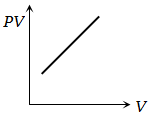2.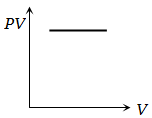3.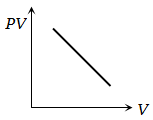4.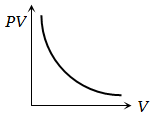High Yielding Test Series + Question Bank - NEET 2020

Difficulty Level:

The curve between absolute temperature and ${{\mathrm{v}}^{2}}_{\mathrm{rms}}$ is

1.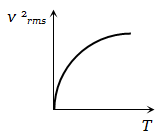2.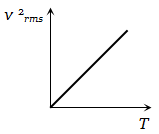3.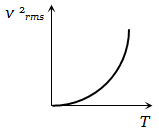4.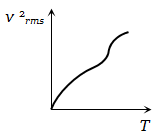High Yielding Test Series + Question Bank - NEET 2020

Difficulty Level:

Volume-temperature graph at atmospheric pressure for a monoatomic gas  is

1.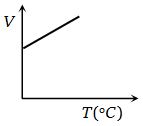2.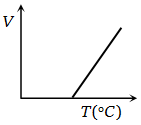3.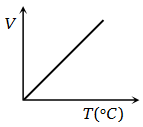4.